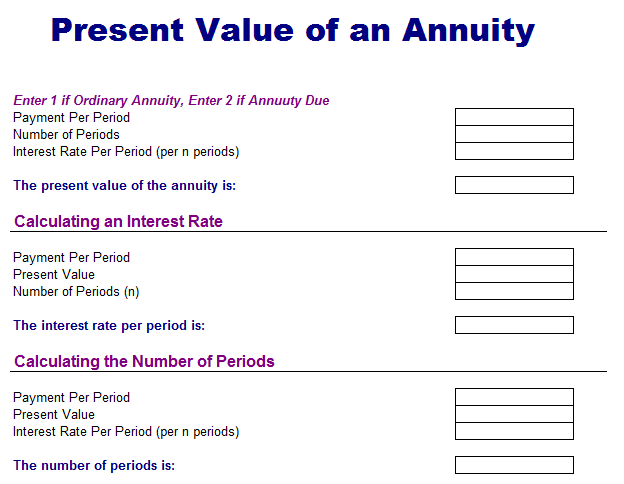# Present Value of Annuity Analysis Template

Annuity is the type of investment where the investor has to pay or receive money in set of different intervals. Sum of money a person receives is normally remains constant over the whole life of annuity. Annuity is considered as stream of cash flows paid out of monthly of annual basis. You can invest in annuity through a bank or financial institution after depositing a lump sum payment to the institution in exchange if series if cash flows paid out over time. The amount of lump sum is considered as the present value of annuity. You can easily calculate the amount of payment needed by taking the future value of annuity. For instance, if you want to invest money for the next 25 years for your retirement with the intention of getting \$150,000 at the time of retirement. You can earn 5 percent interest of the retirement account.

Here is preview of this free Present Value of Annuity Analysis Template created using MS Excel,Time Value of Money (TVM)

Financial sayings are really different from English or moral proverbs. In finance, there is a famous line about financing, “A bird in hand is worth more than a bird in bush”. This is really simple to understand that a bird in bush can easily flyaway at any time leaving you empty handed. Time value of money is an important concept in finance as it will tell you that the value of dollar can be worth more in future than today therefore you have to invest on the higher rate of interest than the rate of inflation. Time value of money is based on finance.

Future Prediction

Every fund manager want to predict future before time and it is possible now as you can get calculated the price of particular stock or bond in the future. It is a certain process to know the investment of your value and fund managers usually forecast the future of markets after considering some certain factors that can influence the direction of investments.

Annuity Factors

Present value of an annuity is calculated by multiplying the cash flows by an annuity factor. Current interest rate and total time period for which the investor will receive the cash flow in future is used as annuity factor. Generally, projected interest rate will determine the value of cash flows such as higher projected interest rate means high present value of cash flows. Lengthy duration for the cash flows will increase the present value of cash flows.

Practical Example

There are different present value annuity tables that will help you to determine the pre-calculated annuity factors. If you want to know the present value of \$500 payments invested at the rate of 8% interest rate in five years, the annuity factor is 19.9636. This shows that the present value of \$500 for 5 years is \$100 x 19.9636 or \$1996.36.  If the same amount is provided for 50 years at the 8% interest rate then the annuity factor will be 61.1674 x \$100 is equal to \$6116.74.